# Java Program to Find Length of Array

In this tutorial you will learn about the Java Program to Find Length of Array and its application with practical example.

## Java Program to Find Length of Array

In this tutorial, we will learn to create a Java program that will Find the Length of an Array in Java programming.

## Prerequisites

Before starting with this tutorial, we assume that you are the best aware of the following Java programming topics:

• Operators in Java Programming.
• Basic Input and Output function in Java Programming.
• Basic Java programming.
• For loop in Java programming.
• Conditional Statements in Java programming.
• Array in Java programming.

## What is An array?

The array is a collection of similar data types. An array can store multiple values with different indexes in memory by using a single variable. The array can be both single and multidimensional.

## Find Length or Size of an Array:-

As we all know, the array is a collection of similar data type elements. In an array, only one variable is declared which can store multiple values. First, will take the number of elements of an array from the user. Then will Find the Length or Size of an Array. And at last, we will print the Length or Size of an Array.

With the help of this program, we can print the Length or Size of an Array.

## Output:-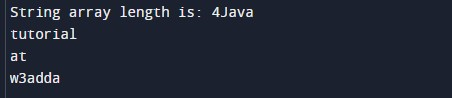In the above program, we have first initialized the required variable.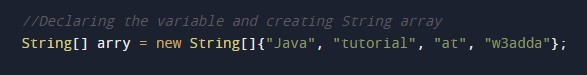• i = it will hold the integer value.
• arr[] = it will hold the integer value.

Finding the length of the array.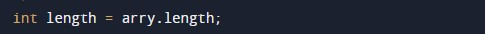Printing the length of the array.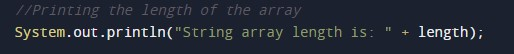Printing the elements of the array.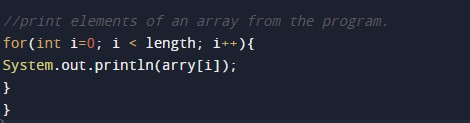In this tutorial we have learn about the Java Program to Find Length of Array and its application with practical example. I hope you will like this tutorial.